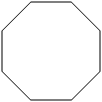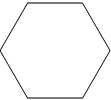Skill 23D 2-Dimensional Figures: Polygons Choose the name for each shape. Then write the number of sides and the number of angles for each shape. polygon name number of sides number of anglespentagon hexagon octagonpentagon hexagon octagonpentagon hexagon octagon